# 词向量

73

## 背景介绍

One-hot vector虽然自然，但是用处有限。比如，在互联网广告系统里，如果用户输入的query是“母亲节”，而有一个广告的关键词是“康乃馨”。虽然按照常理，我们知道这两个词之间是有联系的——母亲节通常应该送给母亲一束康乃馨；但是这两个词对应的one-hot vectors之间的距离度量，无论是欧氏距离还是余弦相似度(cosine similarity)，由于其向量正交，都认为这两个词毫无相关性。 得出这种与我们相悖的结论的根本原因是：每个词本身的信息量都太小。所以，仅仅给定两个词，不足以让我们准确判别它们是否相关。要想精确计算相关性，我们还需要更多的信息——从大量数据里通过机器学习方法归纳出来的知识。

$$X = USV^T$$

1) 由于很多词没有出现，导致矩阵极其稀疏，因此需要对词频做额外处理来达到好的矩阵分解效果；
2) 矩阵非常大，维度太高(通常达到$10^6*10^6$的数量级)；
3) 需要手动去掉停用词（如although, a,...），不然这些频繁出现的词也会影响矩阵分解的效果。

## 效果展示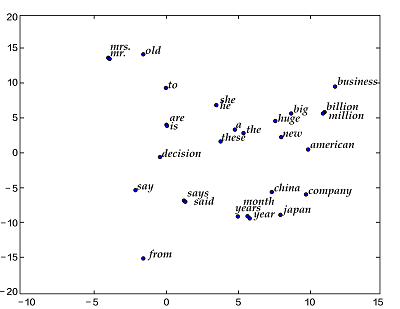similarity: 0.899180685161
please input two words: big huge

please input two words: from company
similarity: -0.0997506977351

## 模型概览

### 语言模型

$$P(w_1, ..., w_T) = \prod_{t=1}^TP(w_t)$$

$$P(w_1, ..., w_T) = \prod_{t=1}^TP(w_t | w_1, ... , w_{t-1})$$

### N-gram neural model

Yoshua Bengio等科学家就于2003年在著名论文 Neural Probabilistic Language Models  中介绍如何学习一个神经元网络表示的词向量模型。文中的神经概率语言模型（Neural Network Language Model，NNLM）通过一个线性映射和一个非线性隐层连接，同时学习了语言模型和词向量，即通过学习大量语料得到词语的向量表达，通过这些向量得到整个句子的概率。用这种方法学习语言模型可以克服维度灾难（curse of dimensionality）,即训练和测试数据不同导致的模型不准。注意：由于“神经概率语言模型”说法较为泛泛，我们在这里不用其NNLM的本名，考虑到其具体做法，本文中称该模型为N-gram neural model。

$$P(w_1, ..., w_T) = \prod_{t=n}^TP(w_t|w_{t-1}, w_{t-2}, ..., w_{t-n+1})$$

$$\frac{1}{T}\sum_t f(w_t, w_{t-1}, ..., w_{t-n+1};\theta) + R(\theta)$$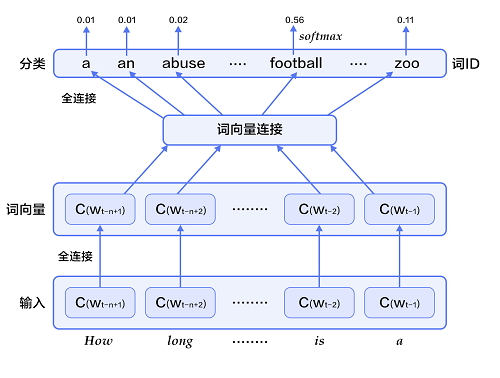每个输入词$w_{t-n+1},...w_{t-1}$首先通过映射矩阵映射到词向量$C(w_{t-n+1}),...C(w_{t-1})$。

然后所有词语的词向量连接成一个大向量，并经过一个非线性映射得到历史词语的隐层表示：

$$g=Utanh(\theta^Tx + b_1) + Wx + b_2$$

根据softmax的定义，通过归一化$g_i$, 生成目标词$w_t$的概率为：

$$P(w_t | w_1, ..., w_{t-n+1}) = \frac{e^{g_{w_t}}}{\sum_i^{|V|} e^{g_i}}$$

整个网络的损失值(cost)为多类分类交叉熵，用公式表示为

$$J(\theta) = -\sum_{i=1}^N\sum_{c=1}^{|V|}y_k^{i}log(softmax(g_k^i))$$

其中$y_k^i$表示第$i$个样本第$k$类的真实标签(0或1)，$softmax(g_k^i)$表示第i个样本第k类softmax输出的概率。

### Continuous Bag-of-Words model(CBOW)

CBOW模型通过一个词的上下文（各N个词）预测当前词。当N=2时，模型如下图所示：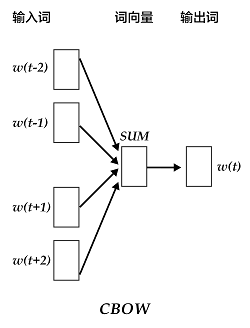$$context = \frac{x_{t-1} + x_{t-2} + x_{t+1} + x_{t+2}}{4}$$

### Skip-gram model

CBOW的好处是对上下文词语的分布在词向量上进行了平滑，去掉了噪声，因此在小数据集上很有效。而Skip-gram的方法中，用一个词预测其上下文，得到了当前词上下文的很多样本，因此可用于更大的数据集。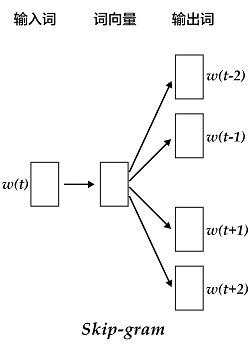## 数据准备

### 数据预处理

<s> I have a dream
I have a dream that
have a dream that one
a dream that one day
dream that one day <e>

## 编程实现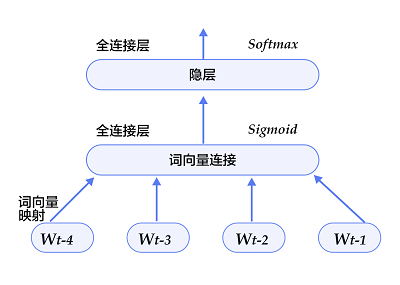import math
import paddle.v2 as paddle

embsize = 32 # 词向量维度
hiddensize = 256 # 隐层维度
N = 5 # 训练5-Gram

将$w_t$之前的$n-1$个词 $w_{t-n+1},...w_{t-1}$，通过$|V|\times D$的矩阵映射到D维词向量（本例中取D=32）。

def wordemb(inlayer):
wordemb = paddle.layer.table_projection(
input=inlayer,
size=embsize,
param_attr=paddle.attr.Param(
name="_proj",
initial_std=0.001,
learning_rate=1,
l2_rate=0,
sparse_update=True))
return wordemb

定义输入层接受的数据类型以及名字。

paddle.init(use_gpu=False, trainer_count=3) # 初始化PaddlePaddle
word_dict = paddle.dataset.imikolov.build_dict()
dict_size = len(word_dict)
# 每个输入层都接受整形数据，这些数据的范围是[0, dict_size)
firstword = paddle.layer.data(
name="firstw", type=paddle.data_type.integer_value(dict_size))
secondword = paddle.layer.data(
name="secondw", type=paddle.data_type.integer_value(dict_size))
thirdword = paddle.layer.data(
name="thirdw", type=paddle.data_type.integer_value(dict_size))
fourthword = paddle.layer.data(
name="fourthw", type=paddle.data_type.integer_value(dict_size))
nextword = paddle.layer.data(
name="fifthw", type=paddle.data_type.integer_value(dict_size))

Efirst = wordemb(firstword)
Esecond = wordemb(secondword)
Ethird = wordemb(thirdword)
Efourth = wordemb(fourthword)

将这n-1个词向量经过concat_layer连接成一个大向量作为历史文本特征。

contextemb = paddle.layer.concat(input=[Efirst, Esecond, Ethird, Efourth])

将历史文本特征经过一个全连接得到文本隐层特征。

hidden1 = paddle.layer.fc(input=contextemb,
size=hiddensize,
act=paddle.activation.Sigmoid(),
layer_attr=paddle.attr.Extra(drop_rate=0.5),
bias_attr=paddle.attr.Param(learning_rate=2),
param_attr=paddle.attr.Param(
initial_std=1. / math.sqrt(embsize * 8),
learning_rate=1))

将文本隐层特征，再经过一个全连接，映射成一个$|V|$维向量，同时通过softmax归一化得到这|V|个词的生成概率。

predictword = paddle.layer.fc(input=hidden1,
size=dict_size,
bias_attr=paddle.attr.Param(learning_rate=2),
act=paddle.activation.Softmax())

网络的损失函数为多分类交叉熵，可直接调用classification_cost函数。

cost = paddle.layer.classification_cost(input=predictword, label=nextword)

训练方法（optimizer)： 代表训练过程在更新权重时采用动量优化器，本教程使用Adam优化器。
训练速度（learning_rate）： 迭代的速度，与网络的训练收敛速度有关系。
正则化（regularization）： 是防止网络过拟合的一种手段，此处采用L2正则化。

parameters = paddle.parameters.create(cost)
adagrad = paddle.optimizer.AdaGrad(
learning_rate=3e-3,
regularization=paddle.optimizer.L2Regularization(8e-4))
trainer = paddle.trainer.SGD(cost, parameters, adagrad)

paddle.batch的输入是一个reader，输出是一个batched reader —— 在PaddlePaddle里，一个reader每次yield一条训练数据，而一个batched reader每次yield一个minbatch。

import gzip

def event_handler(event):
if isinstance(event, paddle.event.EndIteration):
if event.batch_id % 100 == 0:
print "Pass %d, Batch %d, Cost %f, %s" % (
event.pass_id, event.batch_id, event.cost, event.metrics)

if isinstance(event, paddle.event.EndPass):
result = trainer.test(
paddle.batch(
paddle.dataset.imikolov.test(word_dict, N), 32))
print "Pass %d, Testing metrics %s" % (event.pass_id, result.metrics)
with gzip.open("model_%d.tar.gz"%event.pass_id, 'w') as f:
parameters.to_tar(f)

trainer.train(
paddle.batch(paddle.dataset.imikolov.train(word_dict, N), 32),
num_passes=100,
event_handler=event_handler)
Pass 0, Batch 0, Cost 7.870579, {'classification_error_evaluator': 1.0}, Testing metrics {'classification_error_evaluator': 0.999591588973999}
Pass 0, Batch 100, Cost 6.136420, {'classification_error_evaluator': 0.84375}, Testing metrics {'classification_error_evaluator': 0.8328699469566345}
Pass 0, Batch 200, Cost 5.786797, {'classification_error_evaluator': 0.8125}, Testing metrics {'classification_error_evaluator': 0.8328542709350586}
...

## 应用模型

### 查看词向量

PaddlePaddle训练出来的参数可以直接使用parameters.get()获取出来。例如查看单词apple的词向量，即为

embeddings = parameters.get("_proj").reshape(len(word_dict), embsize)

print embeddings[word_dict['apple']]